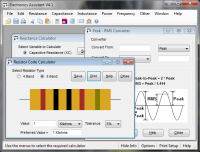# Capacitor Charge / Energy Calculator

The calculator can find the charge (expressed in coulombs) and energy (expressed in joules) stored in a capacitor. Enter the voltage across the capacitor and the capacitance of it. The charge and energy will be shown on the right. The formulae used in the calculations can be found here in the technical data section.

#### Formulae:

Charge: Q = CV where C is the capacitance in Farads, V is the voltage across the capacitor in Volts and Q is the charge measured in coulombs (C).

Energy stored: W = ½ QV = ½ CV2 where W is the energy measured in Joules.

# If you found this calulator useful you should try Electronics Assistant!• All the online calculators and more in a stand-alone application
• Converts Resistor & Inductor colour codes, calculates LED series resistors, capacitance units, series / parallel resistors & capacitors, frequency, reactance & more
• Calculation of nearest preferred resistor values with a choice of 5 series from E12 to E192
• Print & save calculation results